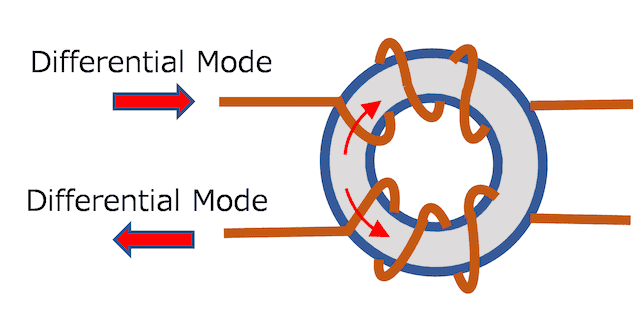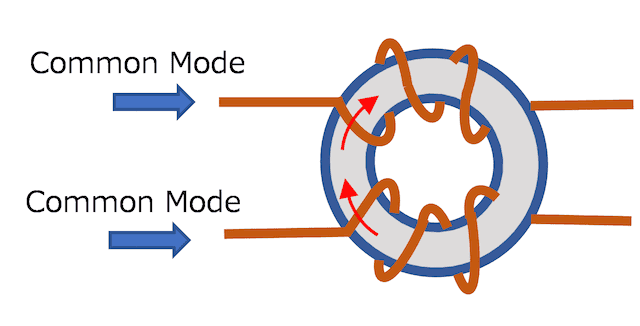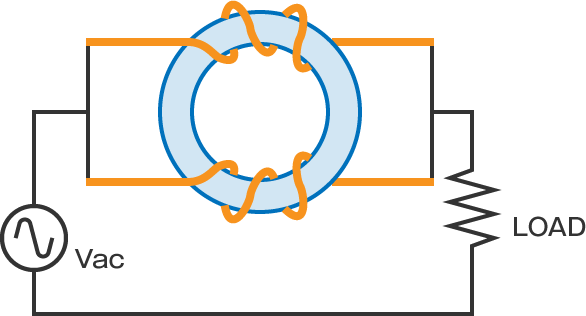### What are Common Mode Choke Coils?

On a Common Mode Choke Coil, the ratio of turns around the primary and secondary windings are equal(L1=L2), and the polarity of the windings (the direction of the windings) are the same. Therefore, the coil functions differently depending on the direction of the magnetic field,  caused by differential and common mode noise currents.

【Effects on Differential Mode Noise】
 In differential mode, the magnetic fields in the toroidal core face opposing directions and cancel out, so it does not act as a coil.  According to this principle, even when a large current flows through the coil in differential mode, the core still acts as a Common Mode Choke Coil without magnetic saturation.【Effects on Common Mode Noise】
 In common mode, the magnetic fields in the toroidal core are facing the same direction, and they strengthen each other, acting as a coil.【Basic Filter Circuit using Common Mode Choke Coil】
As shown in the figure below, you can confirm the function of a Low-pass Filter (LPF) if you check the transmission characteristics of an AC signal input of the same phase between the input/output terminals.Here, the Cut-off Frequency (Fc) when the Load Resistance (LOAD) = R [Ω] can be expressed by the following formula.
Therefore, the inductance value of the Common Mode Coil can be calculated if the cut-off frequency and load resistance are known. The circuit configuration in the above figure is a Low-pass Filter with an attenuation of -20dB/Dec. by LR. The lower the cut-off frequency, the larger the attenuation rate of high-frequency noise.